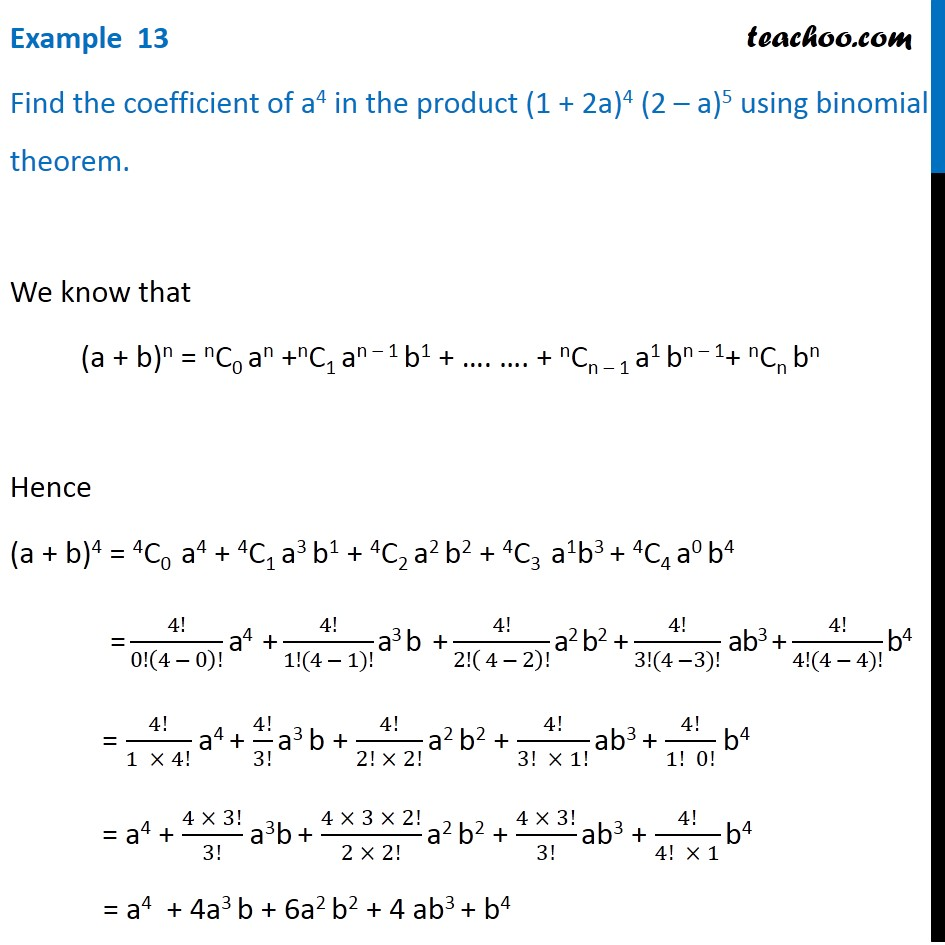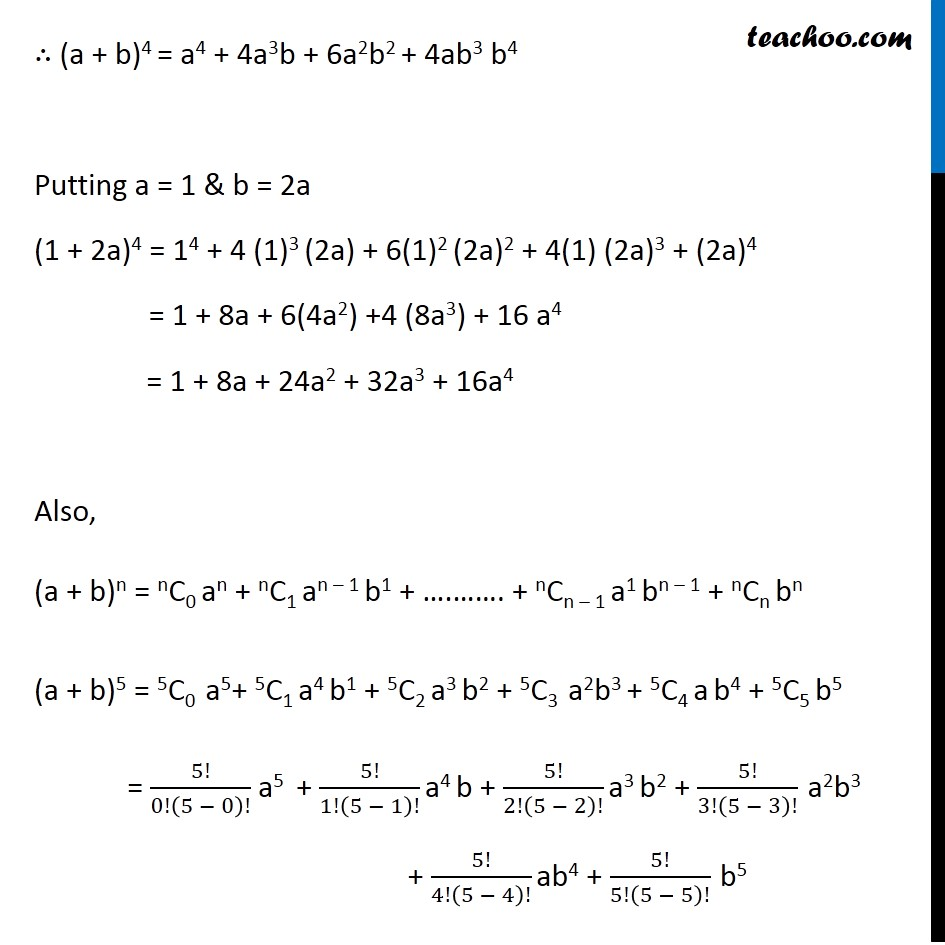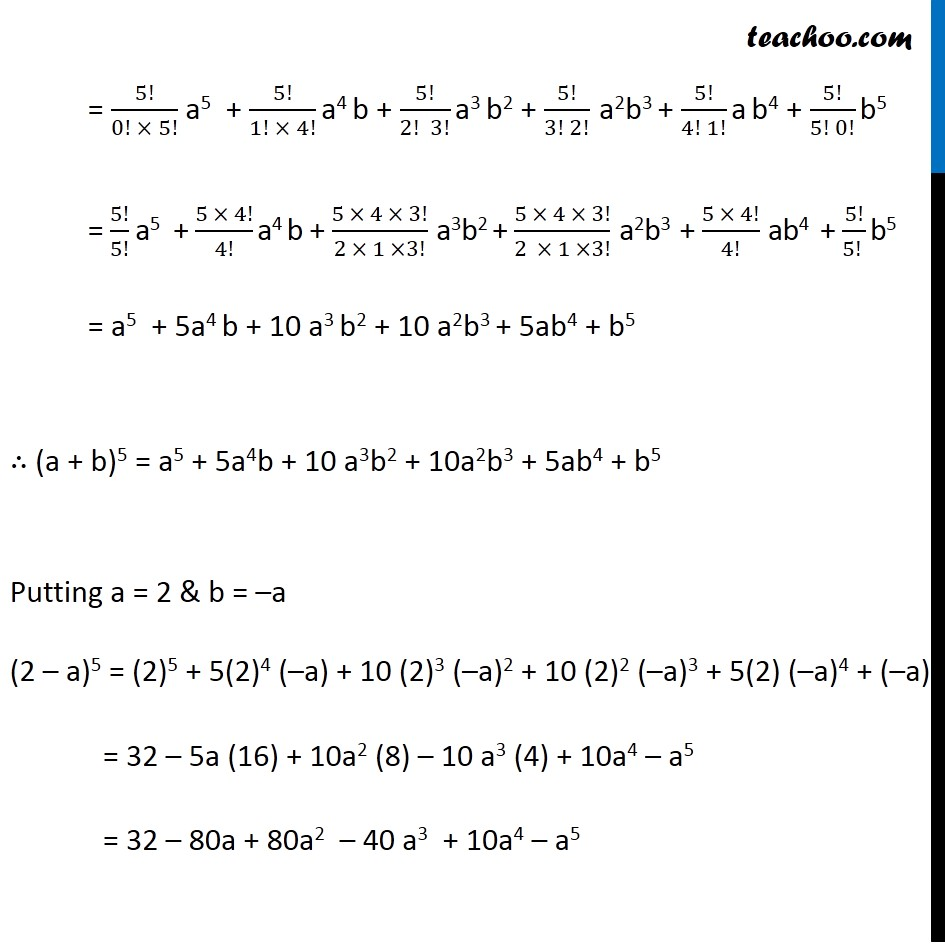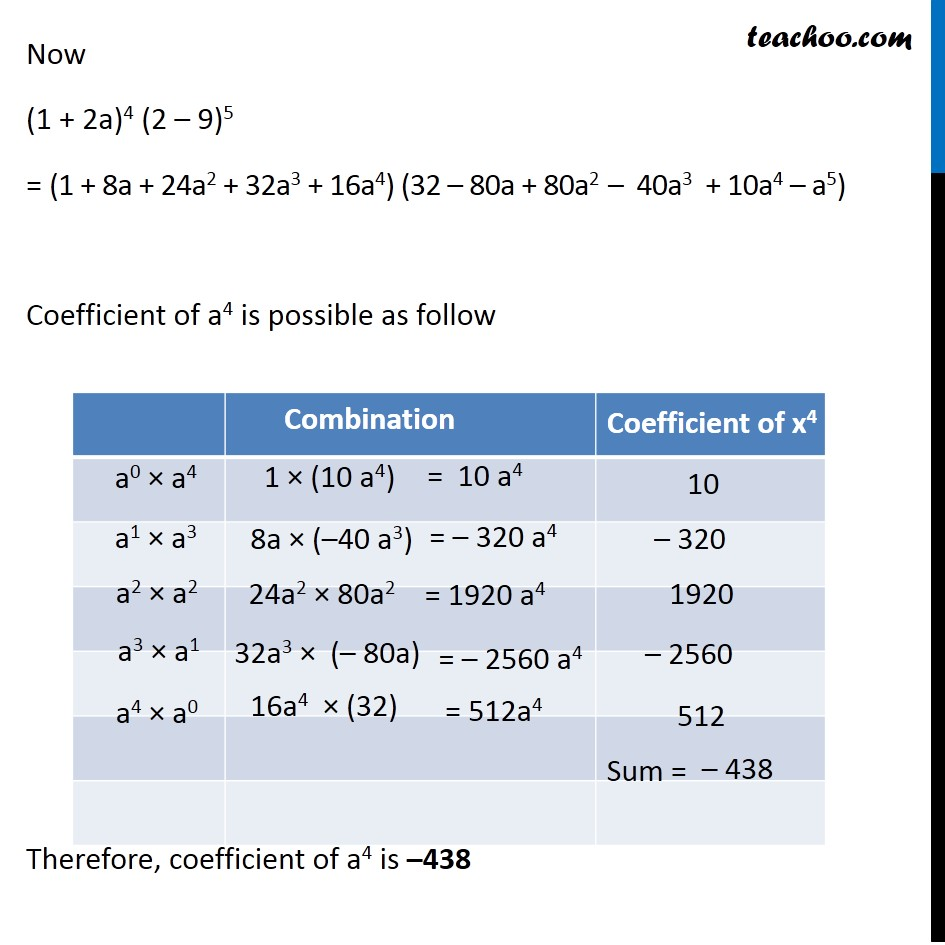1. Chapter 8 Class 11 Binomial Theorem (Deleted)
2. Serial order wise
3. Examples

Transcript

Example 13 Find the coefficient of a4 in the product (1 + 2a)4 (2 – a)5 using binomial theorem. We know that (a + b)n = nC0 an +nC1 an – 1 b1 + …. …. + nCn – 1 a1 bn – 1+ nCn bn Hence (a + b)4 = 4C0 a4 + 4C1 a3 b1 + 4C2 a2 b2 + 4C3 a1b3 + 4C4 a0 b4 = 4!/0!(4 − 0)! a4 + 4!/(1!(4 − 1)!) a3 b + 4!/2!( 4 − 2)! a2 b2 + 4!/(3!(4 −3)!) ab3 + 4!/(4!(4 − 4)!) b4 = 4!/(1 × 4!) a4 + 4!/3! a3 b + 4!/(2! × 2!) a2 b2 + 4!/(3! × 1!) ab3 + 4!/(1! 0!) b4 = a4 + (4 × 3!)/3! a3b + (4 × 3 × 2!)/(2 × 2!) a2 b2 + (4 × 3!)/3! ab3 + 4!/(4! × 1) b4 = a4 + 4a3 b + 6a2 b2 + 4 ab3 + b4 ∴ (a + b)4 = a4 + 4a3b + 6a2b2 + 4ab3 b4 Putting a = 1 & b = 2a (1 + 2a)4 = 14 + 4 (1)3 (2a) + 6(1)2 (2a)2 + 4(1) (2a)3 + (2a)4 = 1 + 8a + 6(4a2) +4 (8a3) + 16 a4 = 1 + 8a + 24a2 + 32a3 + 16a4 Also, (a + b)n = nC0 an + nC1 an – 1 b1 + ….……. + nCn – 1 a1 bn – 1 + nCn bn (a + b)5 = 5C0 a5+ 5C1 a4 b1 + 5C2 a3 b2 + 5C3 a2b3 + 5C4 a b4 + 5C5 b5 = 5!/0!(5 − 0)! a5 + 5!/1!(5 − 1)! a4 b + 5!/2!(5 − 2)! a3 b2 + 5!/3!(5 − 3)! a2b3 + 5!/4!(5 − 4)! ab4 + 5!/5!(5 − 5)! b5 = 5!/(0! × 5!) a5 + 5!/(1! × 4!) a4 b + 5!/(2! 3!) a3 b2 + 5!/(3! 2!) a2b3 + 5!/(4! 1!) a b4 + 5!/(5! 0!) b5 = 5!/5! a5 + (5 × 4!)/4! a4 b + (5 × 4 × 3!)/(2 × 1 ×3!) a3b2 + (5 × 4 × 3!)/(2 × 1 ×3!) a2b3 + (5 × 4!)/4! ab4 + 5!/(5! ) b5 = a5 + 5a4 b + 10 a3 b2 + 10 a2b3 + 5ab4 + b5 ∴ (a + b)5 = a5 + 5a4b + 10 a3b2 + 10a2b3 + 5ab4 + b5 Putting a = 2 & b = –a (2 – a)5 = (2)5 + 5(2)4 (–a) + 10 (2)3 (–a)2 + 10 (2)2 (–a)3 + 5(2) (–a)4 + (–a)5 = 32 – 5a (16) + 10a2 (8) – 10 a3 (4) + 10a4 – a5 = 32 – 80a + 80a2 – 40 a3 + 10a4 – a5 Now (1 + 2a)4 (2 – 9)5 = (1 + 8a + 24a2 + 32a3 + 16a4) (32 – 80a + 80a2 – 40a3 + 10a4 – a5) Coefficient of a4 is possible as follow Therefore, coefficient of a4 is –438 Hence, the coefficient of a4 in the given product is – 438